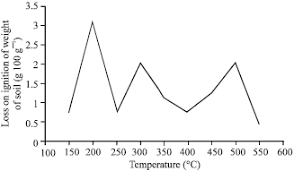## How to Calculate and Solve for Loss on Ignition | Refractories

The loss on ignition is illustrated by the image below.To compute for loss on ignition, two essential parameters are needed and these parameters are Weight before Firing (m1) and Weight after Firing (m2).

The formula for calculating loss on ignition:

LOI = 100((m1 – m2)/m1)

Where:

LOI = Loss On Ignition
m1 = Weight before Firing
m2 = Weight after Firing

Let’s solve an example;
Find the loss on ignition when the weight before firing is 22 and the weight after firing is 11.

This implies that;

m1 = Weight before Firing = 22
m2 = Weight after Firing = 11

LOI = 100((m1 – m2)/m1)
LOI = 100((22 – 11)/22)
LOI = 100(11/22)
LOI = 100(0.5)
LOI = 50

Therefore, the loss on ignition is 50%.